# PyTorch 08：transforms 数据增强：裁剪、翻转、旋转

## PyTorch 学习笔记

Posted by YEY on December 12, 2020

# Lecture 08 transforms 数据增强：裁剪、翻转、旋转

## 1. 数据增强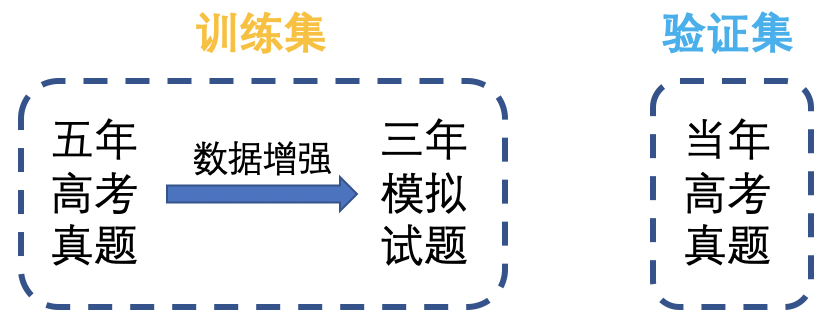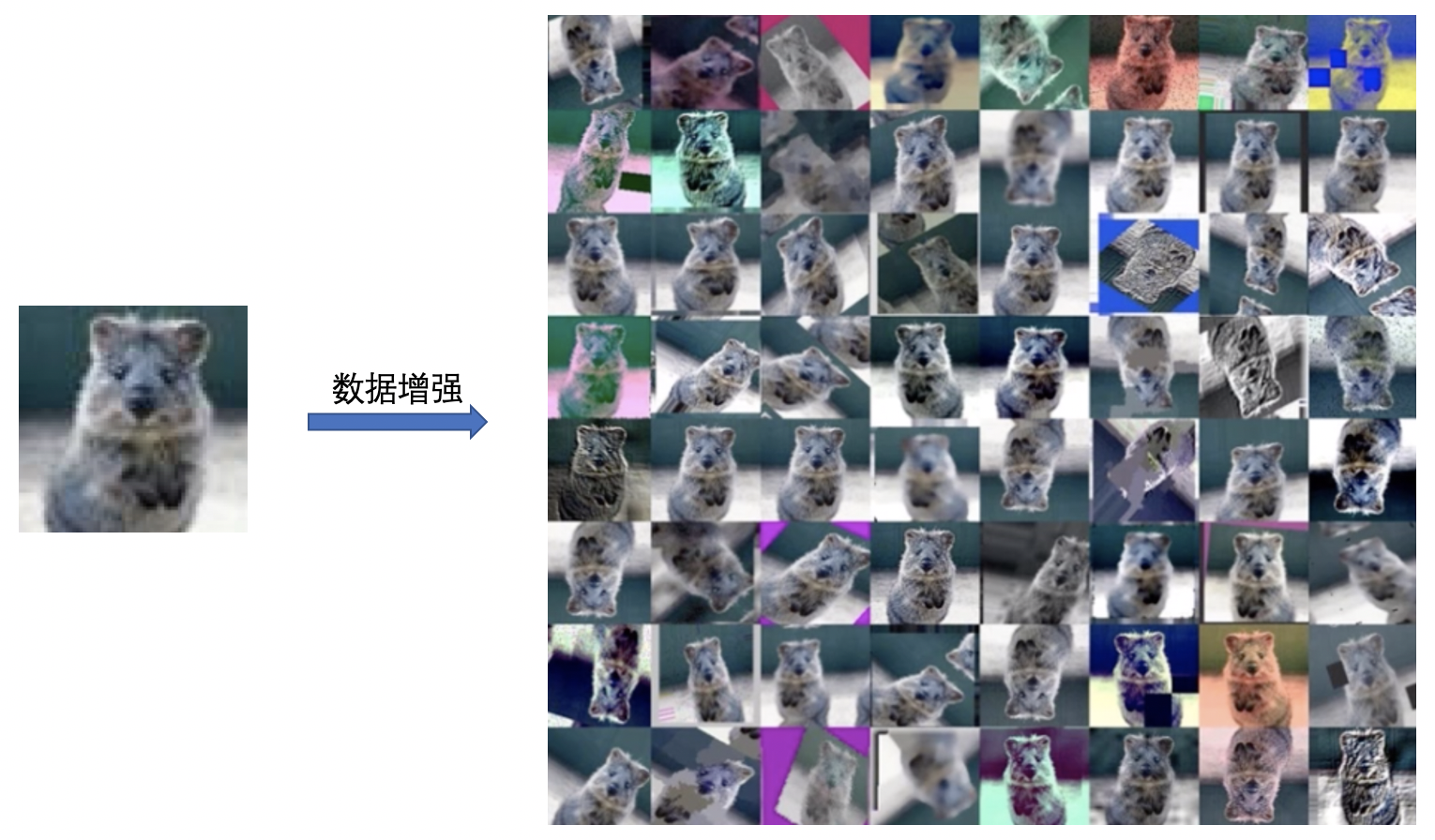## 2. transforms 裁剪

#### transforms.CenterCrop

1
transforms.CenterCrop(size)


• size：所需裁剪图片尺寸。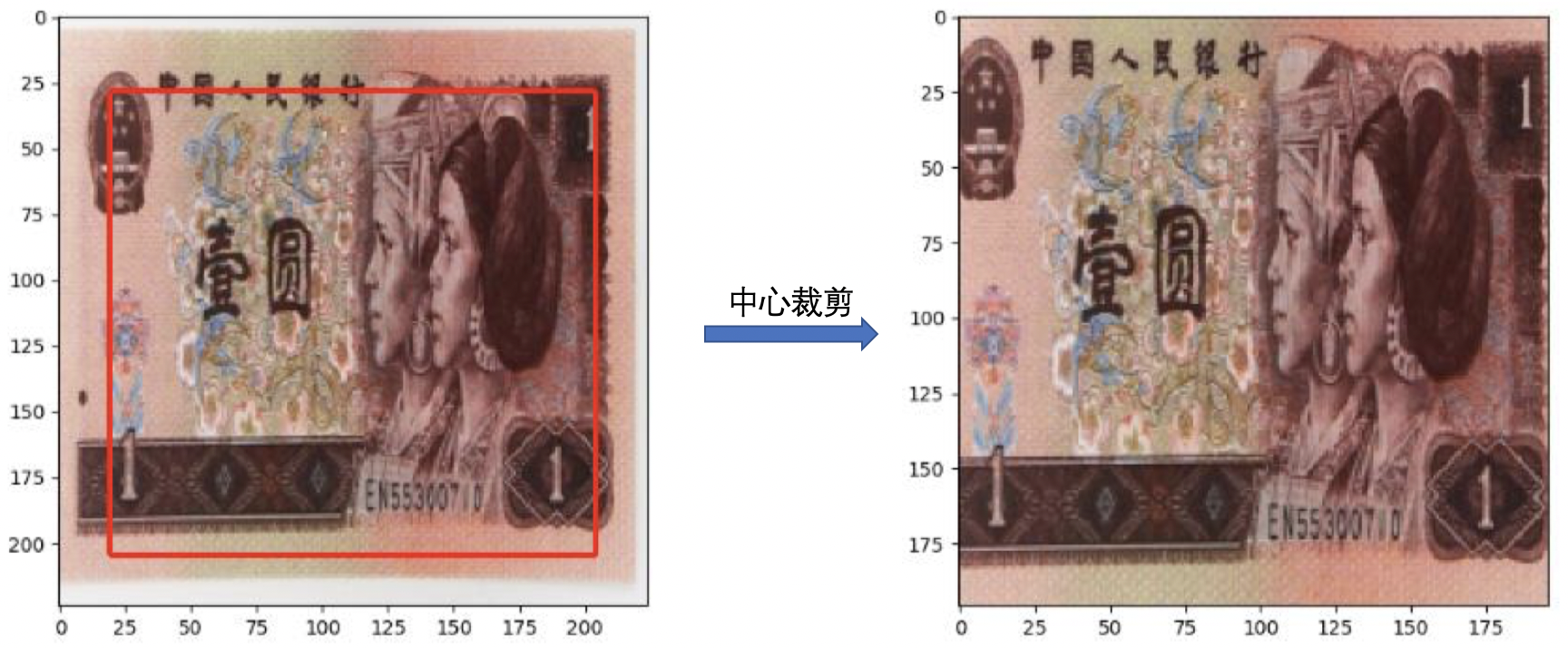1
2
3
4
5
6
train_transform = transforms.Compose([
transforms.Resize((224, 224)),

# CenterCrop，如果 size 大于原始尺寸，多余部分将用黑色 (即像素值为 0) 填充
transforms.CenterCrop(196)
])


#### transforms.RandomCrop

1
2
3
4
5
6
7
transforms.RandomCrop(
size,
fill=0,
)


• size：所需裁剪图片尺寸。
• padding：设置填充大小。
• 当为 a 时，上下左右均填充 a 个像素。
• 当为 (a, b) 时， 上下填充 b 个像素， 左右填充 a 个像素。
• 当为 (a, b, c, d) 时，左、上、右、下分别填充 abcd 个像素。
• pad_if_need：若图像小于设定 size，则填充，此时该项需要设置为 True
• padding_mode：填充模式，有 4 种模式：
• constant：像素值由 fill 设定。
• edge：像素值由图像边缘像素决定。
• reflect：镜像填充，最后一个像素不镜像，例如 [1, 2, 3, 4] $\to$ [3, 2, 1, 2, 3, 4, 3, 2]
• symmetric：镜像填充，最后一个像素镜像，例如 [1, 2, 3, 4] $\to$ [2, 1, 1, 2, 3, 4, 4, 3]
• fillpadding_mode = 'constant' 时，设置填充的像素值。

#### transforms.RandomResizedCrop

1
2
3
4
5
6
RandomResizedCrop(
size,
scale=(0.08, 1.0),
ratio=(3/4, 4/3),
interpolation
)


• size：所需裁剪图片尺寸。
• scale：随机裁剪面积比例，默认 (0.08, 1)
• ratio：随机长宽比，默认 (3/4, 4/3)
• interpolation：插值方法。
• PIL.Image.NEAREST
• PIL.Image.BILINEAR
• PIL.Image.BICUBIC

#### transforms.FiveCrop

1
transforms.FiveCrop(size)


• size：所需裁剪图片尺寸。

1
2
3
4
5
6
train_transform = transforms.Compose([
transforms.Resize((224, 224)),
# 注意：由于生成了 5 张图片，返回的是一个元组，我们需要将其转换为 PIL Image 或者 ndarray 的形式。
transforms.FiveCrop(112),
transforms.Lambda(lambda crops: torch.stack([(transforms.ToTensor()(crop)) for crop in crops]))
])


#### transforms.TenCrop

1
2
3
4
transforms.TenCrop(
size,
vertical_flip=False
)


• size：所需裁剪图片尺寸。
• vertical_flip：是否垂直翻转。

1
2
3
4
5
6
train_transform = transforms.Compose([
transforms.Resize((224, 224)),
# 注意：由于生成了 10 张图片，返回的是一个元组，我们需要将其转换为 PIL Image 或者 ndarray 的形式。
transforms.TenCrop(112, vertical_flip=False),
transforms.Lambda(lambda crops: torch.stack([(transforms.ToTensor()(crop)) for crop in crops])),
])


## 3. transforms 翻转、旋转

### 3.1 transforms 翻转

#### transforms.RandomHorizontalFlip

1
transforms.RandomHorizontalFlip(p=0.5)


• p：翻转概率。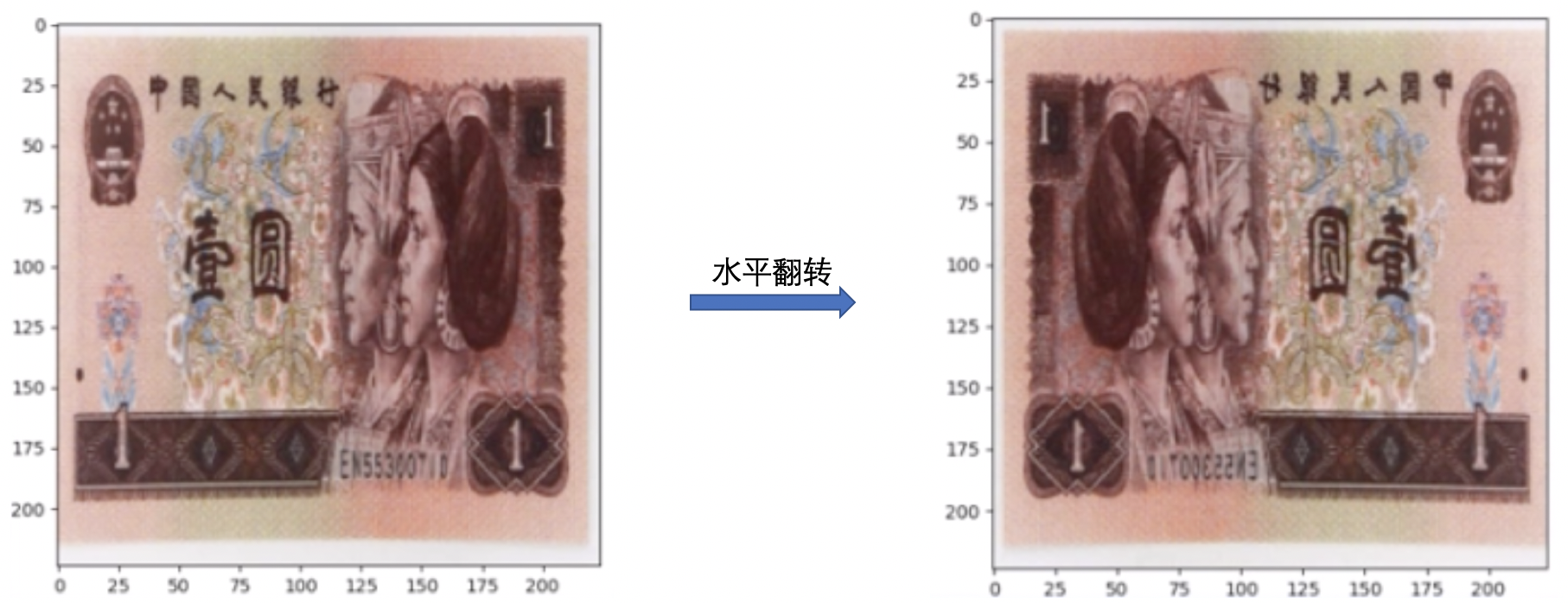#### transforms.RandomVerticalFlip

1
transforms.RandomVerticalFlip(p=0.5)


• p：翻转概率。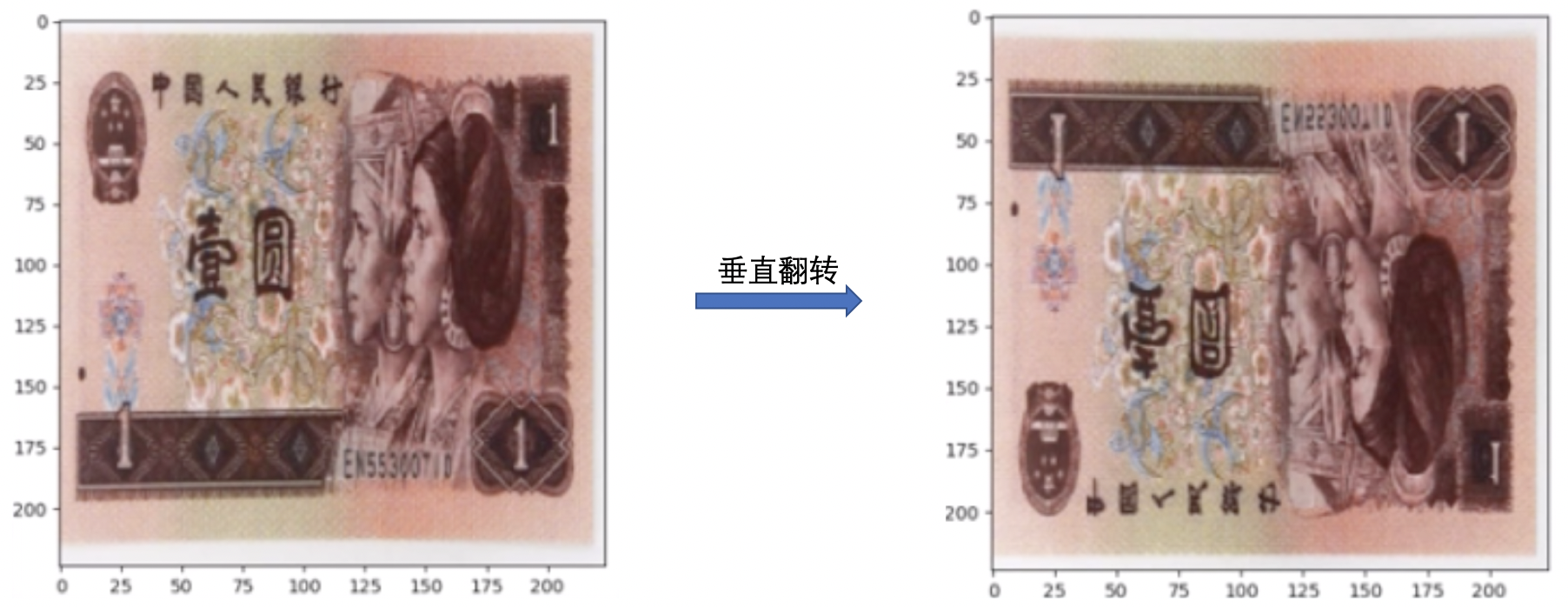### 3.2 transforms 旋转

#### transforms.RandomRotation

1
2
3
4
5
6
RandomRotation(
degrees,
resample=False,
expand=False,
center=None
)


• degrees：旋转角度。
• 当为 a 时，在 (-a, a) 之间随机选择旋转角度。
• 当为 (a, b) 时，在 (a, b) 之间随机选择旋转角度。
• resample：重采样方法。
• expand：是否扩大图片，以保持原图信息。
• center：旋转点设置，默认中心旋转。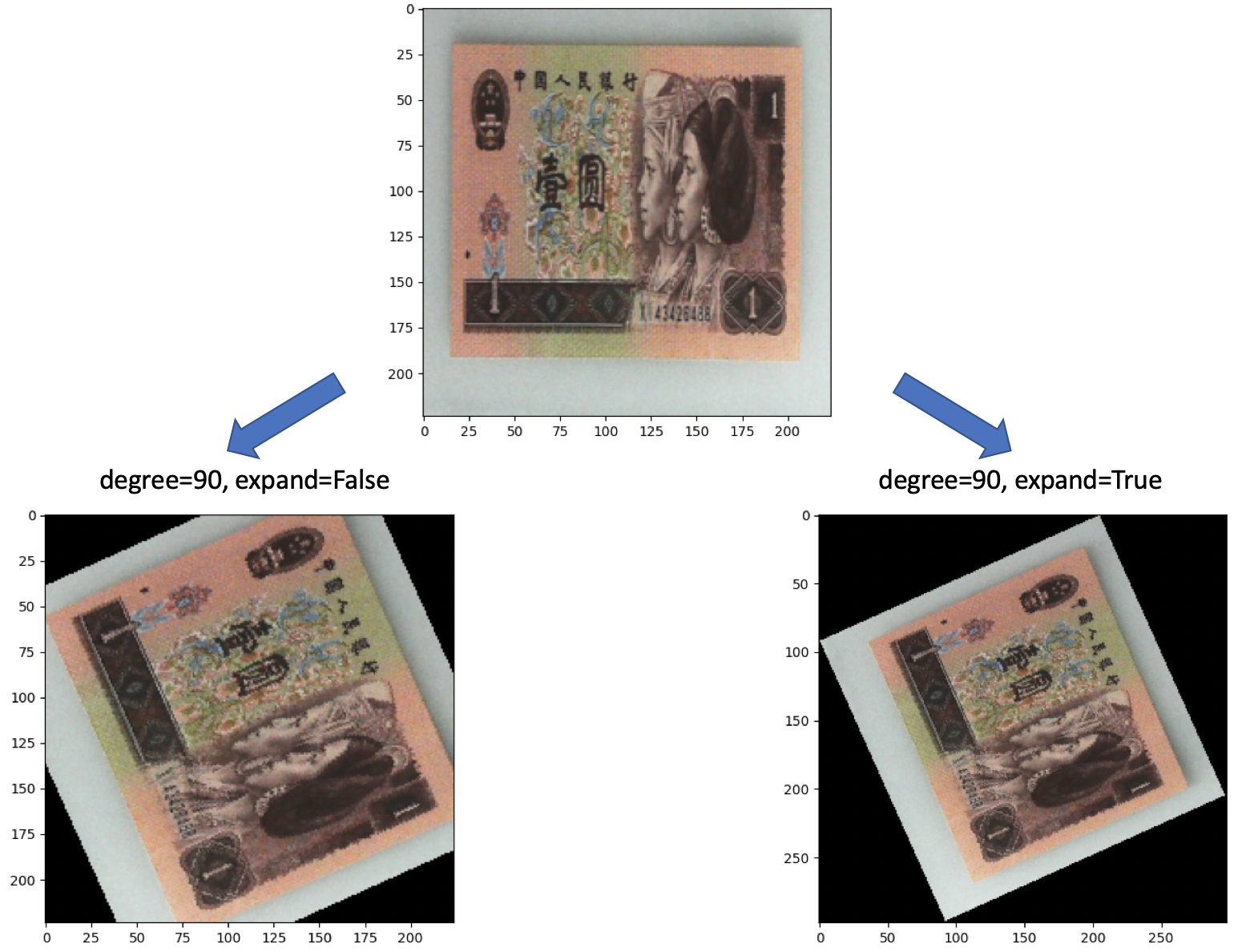## 4. 总结本作品采用知识共享署名-非商业性使用-相同方式共享 4.0 国际许可协议进行许可。 欢迎转载，并请注明来自：YEY 的博客 同时保持文章内容的完整和以上声明信息！